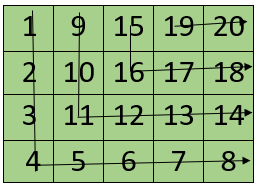# Traverse matrix in L shape

Given a N * M matrix. The task is to traverse the given matrix in L shape as shown in below image.Examples:

```Input : n = 3, m = 3
a[][] = { { 1, 2, 3 },
{ 4, 5, 6 },
{ 7, 8, 9 } }
Output : 1 4 7 8 9 2 5 6 3

Input : n = 3, m = 4
a[][] = { { 1, 2, 3 },
{ 4, 5, 6 },
{ 7, 8, 9 },
{ 10, 11, 12} }
Output : 1 4 7 10 11 12 2 5 8 9 3 6
```

Observe there will be m (number of columns) number of L shapes that need to be traverse. So we will traverse each L shape in two parts, first vertical (top to down) and then horizontal (left to right).
To traverse in vertically, observe for each column j, 0 <= j <= m – 1, we need to traverse n – j elements vertically. So for each column j, traverse from a[j] to a[n-1-j][j].
Now, to traverse horizontally for each L shape, observe the corresponding row for each column j will be (n-1-j)th row and the first element will be (j+1)th element from the beginning of the row. So, for each L shape or for each column j, to traverse horizontally, traverse from a[n-1-j][j+1] to a[n-1-j][m-1].

Below is the implementation of this approach:

## C++

 `// C++ program to traverse a m x n matrix in L shape. ` `#include ` `using` `namespace` `std; ` ` `  `#define MAX 100 ` ` `  `// Printing matrix in L shape ` `void` `traverseLshape(``int` `a[][MAX], ``int` `n, ``int` `m) ` `{ ` `    ``// for each column or each L shape ` `    ``for` `(``int` `j = 0; j < m; j++) { ` ` `  `        ``// traversing vertically ` `        ``for` `(``int` `i = 0; i <= n - j - 1; i++) ` `            ``cout << a[i][j] << ``" "``; ` ` `  `        ``// traverse horizontally ` `        ``for` `(``int` `k = j + 1; k < m; k++) ` `            ``cout << a[n - 1 - j][k] << ``" "``; ` `    ``} ` `} ` ` `  `// Driven Program ` `int` `main() ` `{ ` `    ``int` `n = 4; ` `    ``int` `m = 3; ` `    ``int` `a[][MAX] = { { 1, 2, 3 }, ` `                     ``{ 4, 5, 6 }, ` `                     ``{ 7, 8, 9 }, ` `                     ``{ 10, 11, 12 } }; ` `    ``traverseLshape(a, n, m); ` `    ``return` `0; ` `} `

## Java

 `// Java Program to traverse a m x n matrix in L shape. ` `public` `class` `GFG{ ` ` `  `    ``static` `void` `traverseLshape(``int` `a[][], ``int` `n, ``int` `m) { ` `        ``// for each column or each L shape ` `        ``for` `(``int` `j = ``0``; j < m; j++) { ` ` `  `            ``// traversing vertically ` `            ``for` `(``int` `i = ``0``; i <= n - j - ``1``; i++) ` `                ``System.out.print(a[i][j] + ``" "``); ` ` `  `            ``// traverse horizontally ` `            ``for` `(``int` `k = j + ``1``; k < m; k++) ` `                ``System.out.print(a[n - ``1` `- j][k] + ``" "``); ` `        ``} ` `    ``} ` ` `  `    ``// Driver Code ` `    ``public` `static` `void` `main(String args[]) { ` `        ``int` `n = ``4``;  ` `        ``int` `m = ``3``;  ` `        ``int` `a[][] = { { ``1``, ``2``, ``3` `},  ` `                        ``{ ``4``, ``5``, ``6` `},  ` `                        ``{ ``7``, ``8``, ``9` `},  ` `                        ``{ ``10``, ``11``, ``12` `} };  ` `        ``traverseLshape(a, n, m);  ` `    ``} ` `} `

## Python3

 `# Python3 program to traverse a ` `# m x n matrix in L shape. ` ` `  `# Printing matrix in L shape ` `def` `traverseLshape(a, n, m): ` `     `  `    ``# for each column or each L shape ` `    ``for` `j ``in` `range``(``0``, m): ` ` `  `        ``# traversing vertically ` `        ``for` `i ``in` `range``(``0``, n ``-` `j): ` `            ``print``(a[i][j], end ``=` `" "``); ` ` `  `        ``# traverse horizontally ` `        ``for` `k ``in` `range``(j ``+` `1``, m): ` `            ``print``(a[n ``-` `1` `-` `j][k], end ``=` `" "``); ` ` `  `# Driven Code ` `if` `__name__ ``=``=` `'__main__'``: ` `    ``n ``=` `4``; ` `    ``m ``=` `3``; ` `    ``a ``=` `[[``1``, ``2``, ``3``], ` `         ``[``4``, ``5``, ``6``], ` `         ``[``7``, ``8``, ``9``], ` `         ``[``10``, ``11``, ``12``]]; ` `    ``traverseLshape(a, n, m); ` ` `  `# This code is contributed by PrinciRaj1992 `

## C#

 `// C# Program to traverse a m x n matrix in L shape.  ` ` `  `using` `System; ` ` `  `public` `class` `GFG{  ` `   `  `    ``static` `void` `traverseLshape(``int``[,] a, ``int` `n, ``int` `m) {  ` `        ``// for each column or each L shape  ` `        ``for` `(``int` `j = 0; j < m; j++) {  ` `   `  `            ``// traversing vertically  ` `            ``for` `(``int` `i = 0; i <= n - j - 1; i++)  ` `                ``Console.Write(a[i,j] + ``" "``);  ` `   `  `            ``// traverse horizontally  ` `            ``for` `(``int` `k = j + 1; k < m; k++)  ` `                ``Console.Write(a[n - 1 - j,k] + ``" "``);  ` `        ``}  ` `    ``}  ` `   `  `    ``// Driver Code  ` `    ``public` `static` `void` `Main() {  ` `        ``int` `n = 4;   ` `        ``int` `m = 3;   ` `        ``int``[,] a = { { 1, 2, 3 },   ` `                        ``{ 4, 5, 6 },   ` `                        ``{ 7, 8, 9 },   ` `                        ``{ 10, 11, 12 } };   ` `        ``traverseLshape(a, n, m);   ` `    ``}  ` `}  `

Output:

```1 4 7 10 11 12 2 5 8 9 3 6
```

My Personal Notes arrow_drop_upCheck out this Author's contributed articles.

If you like GeeksforGeeks and would like to contribute, you can also write an article using contribute.geeksforgeeks.org or mail your article to contribute@geeksforgeeks.org. See your article appearing on the GeeksforGeeks main page and help other Geeks.

Please Improve this article if you find anything incorrect by clicking on the "Improve Article" button below.

Article Tags :
Practice Tags :

1

Please write to us at contribute@geeksforgeeks.org to report any issue with the above content.